# Millman Theorem

Millman’s theorem was named after famous electrical engineering professor JACOB MILLMAN who proposed the idea of this theorem. Millman’s theorem acts as a very strong tool in case of simplifying the special type of complex electrical circuit. This theorem is nothing but a combination of Thevenin’s Theorem and Norton’s Theorem. It is very useful theorem to find out voltage across the load and current through the load.This theorem is also called as PARALLEL GENERATOR THEOREM.
Millman’s theorem is applicable to a circuit which may contain only voltage sources in parallel or a mixture of voltage and current sources connected in parallel. Let’s discuss these one by one.

### Circuit consisting only Voltage Sources

Let us have a circuit as shown in below figure a.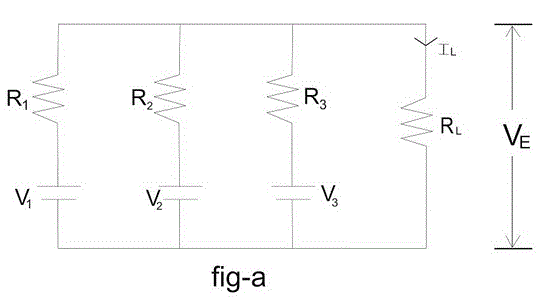Here V1, V2 and V3 are voltages of respectively 1st, 2nd and 3rd branch and R1, R2 and R3 are their respective resistances. IL, RL and VT are load current, load resistance and terminal voltage respectively.
Now this complex circuit can be reduced easily to a single equivalent voltage source with a series resistance with the help of Millman’s Theorem as shown in figure b.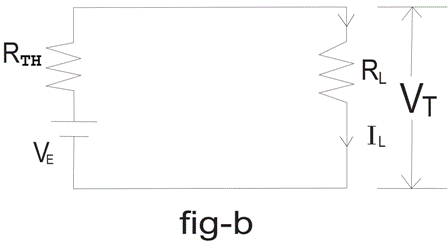The value of equivalent voltage VE is specified as per Millman’s theorem will be –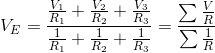This VE is nothing but Thevenin voltage and Thevenin resistance RTH can be determined as per convention by shorting the voltage source. So RTH will be obtained as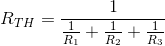Now load current and terminal voltage can be easily found by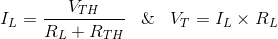Let’s try to understand whole concept of Millman’s Theorem with the help of a example.

Example – 1
A circuit is given as shown in fig-c. Find out the voltage across 2 Ohm resistance and current through the 2 ohm resistance.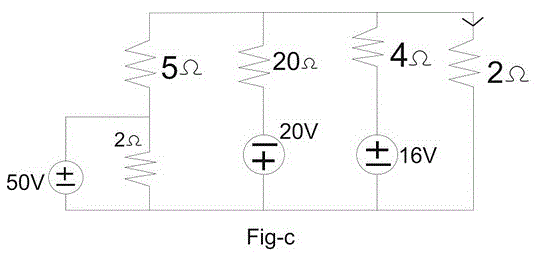Answer : We can go through any solving method to solve this problem but the most effecting and time saving method will be none another than Millman’s theorem. Given circuit can be reduced to a circuit shown in fig-d where equivalent voltage VE can be obtained by millman’s theorem and that is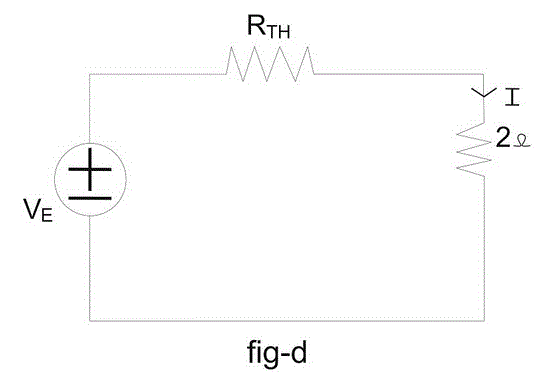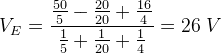Equivalent resistance or Thevenin resistance can be found by shorting the voltage sources as shown in fig – e.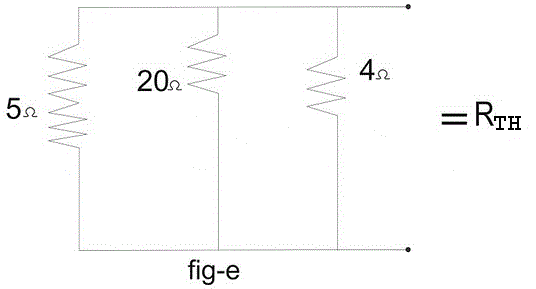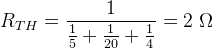Now we can easily found the required current through 2 Ohm load resistance by Ohm’s law.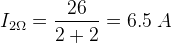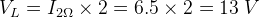### Circuit is Consisting Mixture of Voltage and Current Source

Millman’s Theorem is also helpful to reduce a mixture of voltage and current source connected in parallel to a single equivalent voltage or current source. Let’s have a circuit as shown in below figure – f.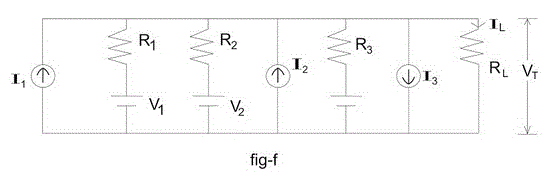Here all letters are implying their conventional representation.This circuit can be reduced to a circuit as shown in figure – g.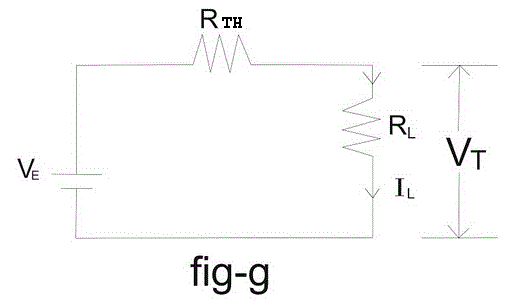Here VE which is nothing but thevenin voltage which will be obtained as per Millman’s theorem and that is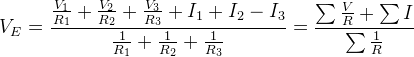And RTH will be obtained by replacing current sources with open circuits and voltage sources with short circuits.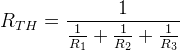Now we can easily find out load current IL and terminal voltage VT by Ohm’s law.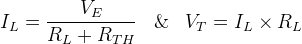Let’s have a example to understand this concept more properly.

Example 2 :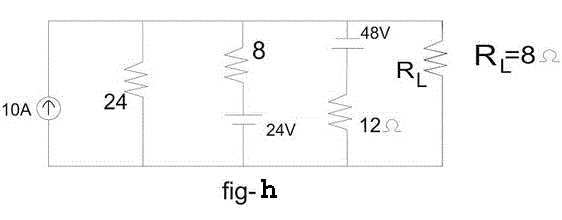A circuit is given as shown in fig-h. Find out the current through load resistance where RL = 8 Ω.

Answer : This problem may seem to be difficult to solve and time consuming but it can easily be solved in a very less time with the help of Millman’s Theorem. The given circuit can be reduced in a circuit as shown in fig – i. Where, VE can be obtained with the help of Millman’s theorem,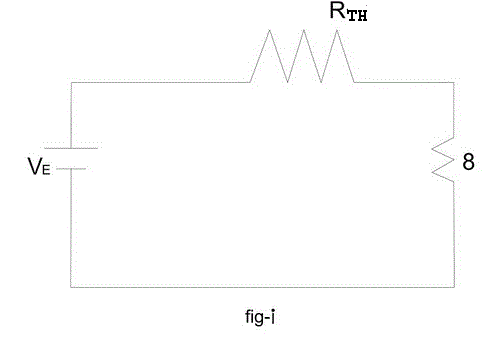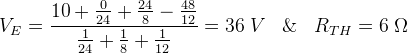Therefore, current through load resistance 8 Ω is,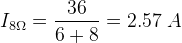Want To Learn Faster? 🎓
Get electrical articles delivered to your inbox every week.
No credit card required—it’s 100% free.